# How to Get Sheet name of worksheet in Excel

In this article, we will learn How to Get Sheet name of worksheet in Excel.

In simple words, If you working with complicated data. And you want your sheet name as text in cell.

CELL Function in Excel gets you the information regarding worksheet like col, contents, filename, ..etc.
Generic formula

=CELL(“filename”,A1)

“filename” : gets the full name of the sheet of the reference cell
A1 : Sheet’s cell reference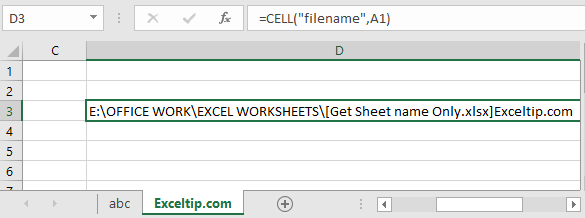But we need to extract just the sheet name. Basically the last name.

As you can see the sheet name starts after ] (closed big bracket sign). For that we just needs its position in the text and then we will extract the sheet name.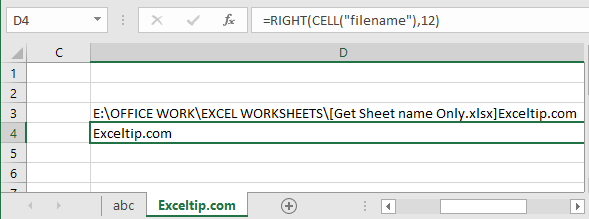Here I counted the characters from the last of the result which was 12. But we cannot do this every time.

So for extracting the sheet name from the full name, we need to find the position of the ] (closed big bracket sign)

Use the formula to find ]

=FIND("]",CELL("filename",A1))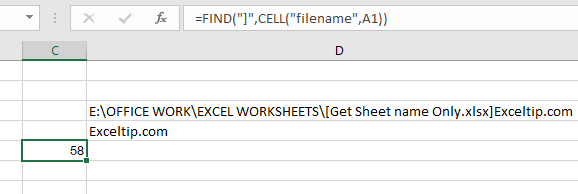58 is the position of ] .

Now our formula is complete.

=MID(CELL("filename",A1),FIND("]",CELL("filename",A1))+1,255)

Explanation:
CELL("filename",A1) : Gets you the full name of the worksheet
FIND("]",CELL("filename",A1))+1 : this function gets you the position of ] and add +1 because we need the position of first char of only sheet name.
255 : Max word limit of sheet name by Excel.
MID : MID Function extracts the specific substring from the text using the position from start to end.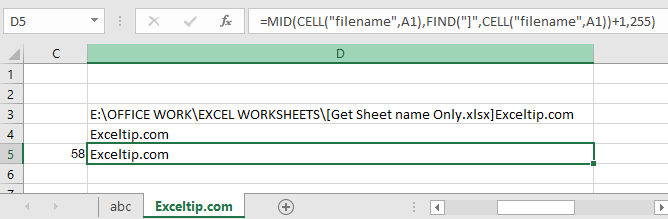Here we extracted the filename using the formula.

Now we have one more formula, instead of using MID function. Use the RIGHT function.
RIGHT function extracts the specific substring from the last of the text.
Use the formula:

=RIGHT(CELL("filename",A2),LEN(CELL("filename",A2))-FIND("]", CELL("filename",A2)))

Explanation:
FIND("]", CELL("filename",A2)) : Extracts the position of sheet name char.
LEN(CELL("filename",A2)) : gets the length of the text(filename)
RIGHT : RIGHT function extracts the string from the text using its position.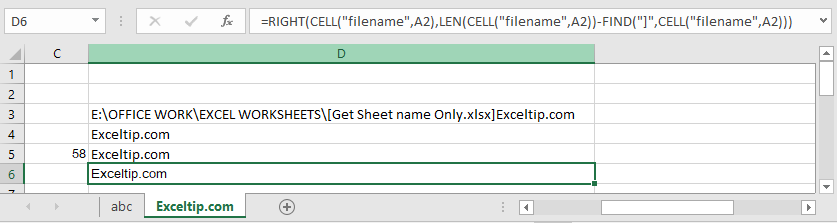As you can see, this function works fine too. Yesss...Now we have 2 different formula with logic used to extract only the sheet name.

Get only the sheet name using VBA in Excel

Hope you understood How to get only sheet name of the worksheet in Excel 2016. Find more articles on Excel TEXT functions here. Please share your query below in the comment box. We will assist you.

Popular Articles

50 Excel Shortcut to Increase Your Productivity : Get faster at your task. These 50 shortcuts will make you work even faster on Excel.

How to use the VLOOKUP Function in Excel : This is one of the most used and popular functions of excel that is used to lookup value from different ranges and sheets.

How to use the COUNTIF function in Excel : Count values with conditions using this amazing function. You don't need to filter your data to count specific values. Countif function is essential to prepare your dashboard.

How to use the SUMIF Function in Excel : This is another dashboard essential function. This helps you sum up values on specific conditions.

Terms and Conditions of use

The applications/code on this site are distributed as is and without warranties or liability. In no event shall the owner of the copyrights, or the authors of the applications/code be liable for any loss of profit, any problems or any damage resulting from the use or evaluation of the applications/code.